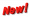Tue. Dec 10th, 2019

# Factoring Worksheets : factorization / find factors of a number#### Factoring is a process in which an expression is converted into a multiplication problem to find its factors. Factors are any number or expression that divides another number or expression evenly, with no remainders. Your students will going to  learn the following factoring skills: factoring the difference of perfect squares; factoring expressions and equations; finding factors of numbers; determining least common multiples and greatest common factors; discovering prime factors, the difference between primes and composites; how to rewrite expressions using factoring; determining common factors in binomial and trinomial expressions; how to use properties such as the distributive; applying factoring to real-world problems.

Factoring is basically a verb that tells us that we need to find the factors of base of the problem we are presented with. In some cases we will be asked to expand number sets, this is the opposite of factoring. When factoring we are often looking for the highest level of commonality between numbers or number sets.

 Current Notifications## Factors

Numbers have factors:And expressions (like x2+4x+3) also have factors:## Factoring

Factoring (called “Factorising” in the UK) is the process of finding the factors:

Factoring: Finding what to multiply together to get an expression.

It is like “splitting” an expression into a multiplication of simpler expressions.

### Example: factor 2y+6

Both 2y and 6 have a common factor of 2:

• 2y is 2 × y
• 6 is 2 × 3

So we can factor the whole expression into:

2y+6 = 2(y+3)

So 2y+6 has been “factored into” 2 and y+3

Factoring is also the opposite of Expanding:## Common Factor

In the previous example we saw that 2y and 6 had a common factor of 2

But to do the job properly we need the highest common factor, including any variables

### Example: factor 3y2+12y

Firstly, 3 and 12 have a common factor of 3.

So we could have:

3y2+12y = 3(y2+4y)

But we can do better!

3y2 and 12y also share the variable y.

Together that makes 3y:

• 3y2 is 3y × y
• 12y is 3y × 4

So we can factor the whole expression into:

3y2+12y = 3y(y+4)

Check: 3y(y+4) = 3y × y + 3y × 4 = 3y2+12y

### Remember these Identities

Here is a list of common “Identities” (including the “difference of squares” used above).

It is worth remembering these, as they can make factoring easier.

 a2 − b2 = (a+b)(a−b) a2 + 2ab + b2 = (a+b)(a+b) a2 − 2ab + b2 = (a−b)(a−b) a3 + b3 = (a+b)(a2−ab+b2) a3 − b3 = (a−b)(a2+ab+b2) a3+3a2b+3ab2+b3 = (a+b)3 a3−3a2b+3ab2−b3 = (a−b)3

There are many more like those, but those are the most useful ones.